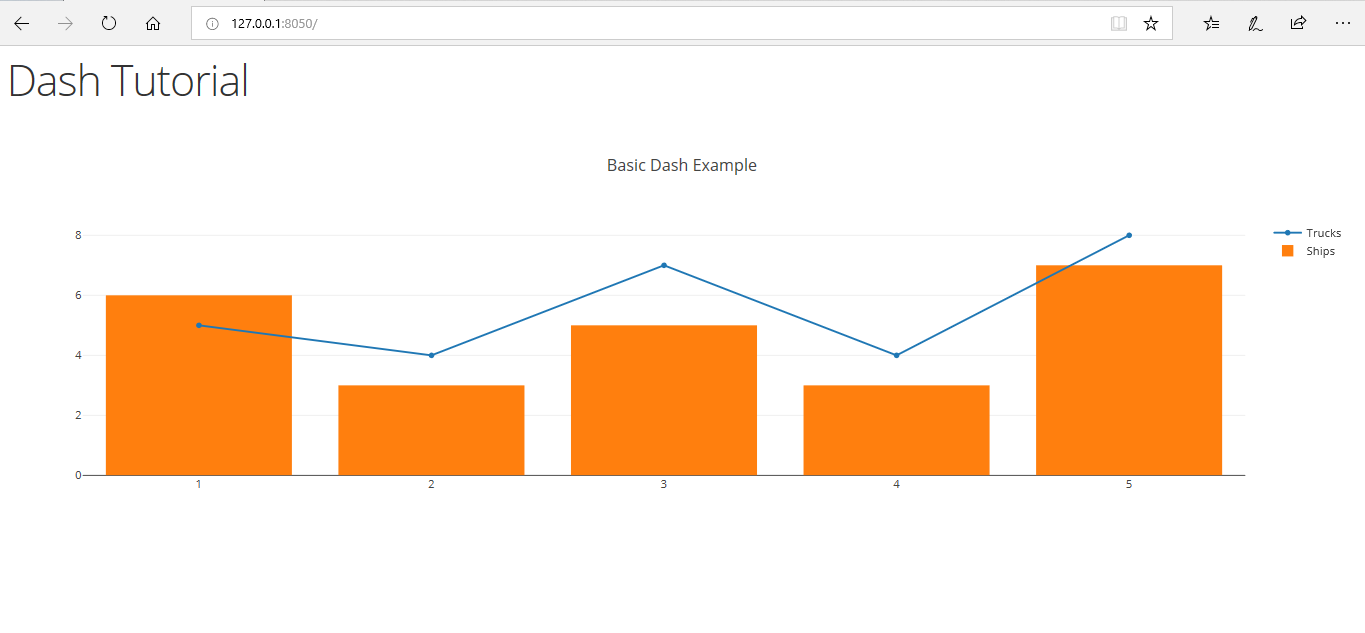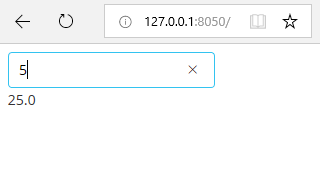# Introduction to Dash in Python

• Difficulty Level : Expert
• Last Updated : 10 May, 2020

Dash is a Python framework for building analytical web applications. Dash helps in building responsive web dashboards that is good to look at and is very fast without the need to understand complex front-end frameworks or languages such as HTML, CSS, JavaScript. Let’s build our first web dashboard using Dash.

## Installation and Imports

```pip install dash
```

## Basic Dashboard

In this section, we will make an app that shows a static(but responsive) graph on the web page using the dash.

Step 1: Importing all the required libraries
Now let’s import Dash, Dash Core Components(which has components like graph, inputs etc., ) and Dash HTML Components(which has HTML components like meta tags, body tags, paragraph tags etc., )

 `# importing required libraries``import` `dash``import` `dash_core_components as dcc    ``import` `dash_html_components as html`

Step 2: Designing a layout
HTML components are just like HTML. Here html.H1 refers to the h1 tag in HTML.
Then, we make a graph which has various parameters such as id(a unique ID to a particular graph), figure(the graph itself), layout(the basic layout, title of graph, X axis, Y axis data etc., ).

• The figure parameter is essentially a dictionary which has elements like x, y, type, name.
• x refers to the X-axis value(it can be a list or a single element), y is the same except it is associated with the Y-axis.
• The type parameter refers to the type of the graph, it maybe line, bar.
• The name parameter refers to the name associated with the axis of a graph
 `app ``=` `dash.Dash()`` ` `app.layout ``=` `html.Div(children ``=``[``    ``html.H1(``"Dash Tutorial"``),``    ``dcc.Graph(``        ``id` `=``"example"``,``        ``figure ``=``{``            ``'data'``:[``                       ``{``'x'``:[``1``, ``2``, ``3``, ``4``, ``5``],``                        ``'y'``:[``5``, ``4``, ``7``, ``4``, ``8``],``                        ``'type'``:``'line'``, ``                        ``'name'``:``'Trucks'``},``                       ``{``'x'``:[``1``, ``2``, ``3``, ``4``, ``5``], ``                        ``'y'``:[``6``, ``3``, ``5``, ``3``, ``7``], ``                        ``'type'``:``'bar'``,``                        ``'name'``:``'Ships'``}``                   ``],``            ``'layout'``:{``                ``'title'``:``'Basic Dashboard'``            ``}``        ``}``    ``)``])`

Step 3: Running the server
The dashboard is now ready, but it needs a server to run on. Thus we set up the server using the below code.

 `if` `__name__ ``=``=` `'__main__'``:``    ``app.run_server()`

Open the app on the web browser in localhost and default port 8050.

```http://127.0.0.1:8050/
```

Output:Screenshot of the Basic Dash app.

## Using Callbacks

The above teaches us a basic static app. But what if you want to let the user take the control. This is why we are going to use app callbacks provided in Dash. In this section, we are going to make a web app that takes in number from the user and return the square of the number

Step 1: Importing all the required libraries
Just like above we are going to import all the required libraries. Here we require an additional dash.dependencies.Input and dash.dependencies.Output to provide us with input and output callback functionality.

 `# importing required libraries``import` `dash``import` `dash_core_components as dcc    ``import` `dash_html_components as html``from` `dash.dependencies ``import` `Input``, Output `

Step 2: Designing a layout

We are going to design a simple textbox that will help out take input and a text label which will output the square of the number that is input or returns an error if the input is not a number.

 `app ``=` `dash.Dash()`` ` `app.layout ``=` `html.Div(children ``=``[``    ``dcc.``Input``(``id` `=``'input'``, ``              ``value ``=``'Enter a number'``, ``              ``type` `=``'text'``),``     ` `    ``html.Div(``id` `=``'output'``)``])`

Step 3: Callbacks

A callback is like a trigger that does a certain function on the change of the state of the input. In this case, it executes the method update_value and takes in input_data as the parameter and returns the square of that number. If the input is not a number, then it returns an error statement.

 `@app``.callback(``    ``Output(component_id ``=``'output'``, component_property ``=``'children'``),``    ``[``Input``(component_id ``=``'input'``, component_property ``=``'value'``)]``)`` ` `def` `update_value(input_data):``    ``try``:``        ``return` `str``(``float``(input_data)``*``*``2``)``    ``except``:``        ``return` `"Error, the input is not a number"`

Step 3: Running the server
Again, just like above, we are going to run the server.

 `if` `__name__ ``=``=` `'__main__'``:``    ``app.run_server()`

Open the app on the web browser in local host and default port 8050.

```http://127.0.0.1:8050/
```

Output:Square of five using Python Dash callbacks.

## Footnotes

The above two examples must be useful for you to understand the working of the Dash framework. Although the two examples written above might not be useful on itself, it does help in understanding the concepts of building web apps with Dash framework and that will help you in building a useful web app in the future using real data.

My Personal Notes arrow_drop_up